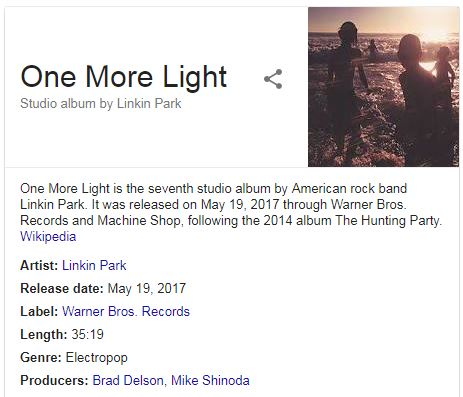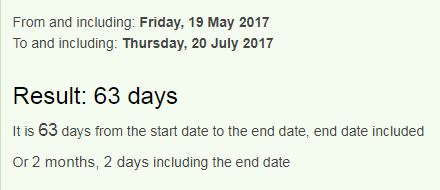## Cipher## Jul 20, 2017

### This "suicide" came by surprise (Chester Bennington)

Today is the 20th of July and we see the news of Chester Bennington committing suicide which is of course a complete bullshit story and it's not really hard to see it hereTake a look at this "symphony of coincidences"
Chester Bennington = 3+8+5+19+20+5+18 + 2+5+14+14+9+14+7+20+15+14 = 192 (English Ordinal)
Twentieth of July = 7+4+22+13+7+18+22+7+19 + 12+21 + 17+6+15+2 = 192 (Reverse Ordinal)
July Twentieth = 10+21+12+25 + 20+23+5+14+20+9+5+20+8 = 192 (English Ordinal)On the 19th of May Linkin Park released their 7th studio album called "One More Light" = 3+4+4 + 5+3+9+4 + 6+9+2+1+7 = 57 (Reverse Reduction)
July Twentieth = 1+3+3+7 + 2+5+5+5+2+9+5+2+8 = 57 (Full Reduction)
On the 63rd day from the release of their album one more light went outIn 2017 his birthday landed on a date with 33 numerology (3/20/2017 = 3+20+2+0+1+7 = 33)
Chester = 3+8+5+1+2+5+9 = 33 (Full Reduction)
Bennington = 25+22+13+13+18+13+20+7+12+13 = 156 (Reverse Ordinal)
One More Light = 15+14+5 + 13+15+18+5 + 12+9+7+8+20 = 141 (English Ordinal)This ritual came after his "seventh" = 8+4+5+4+4+7+1 = 33 (Reverse Reduction) studio album
Of course he committed suicide at the 33rd parallel north and he was born in Phoenix, Arizona located on the same parallelChris Cornell (CC ->33) was found dead on 5/18/2017 a day before "One More Light" was released and today we find Chester dead on Cornell's birthday?Note that he is dead at 41, 4 months and 1 day after his birthday in 2017I don't see any reason to keep throwing numbers around when we have something as clear as this case, if anyone doesn't see it by now nothing is gonna convince them